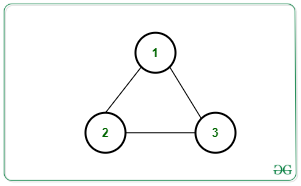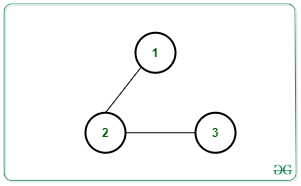Related Articles
Minimum number of edges required to be removed from an Undirected Graph to make it acyclic
• Difficulty Level : Easy
• Last Updated : 18 Sep, 2020

Given an undirected graph consisting of N nodes containing values from the range [1, N] and M edges in a matrix Edges[][], the task is to determine the minimum number of edges required to be removed such that the resulting graph does not contain any cycle.

Examples:

Input: N = 3, M = 3, edges[][] = [[1, 2], [2, 3], [3, 1]]Output: 1
Explanation:
Removing any one of the edges will make the graph acyclic. Therefore, at least one edge needs to be removed.

Input: N = 3, M = 2, edges[][] = [[1, 2], [2, 3]]Output: 0
Explanation: Graph is already acyclic. Therefore, no edge removal is required.

Naive Approach: The simplest approach is to try deleting all possible combination of sequence of edges from the given graph one by one and for each combination, count the number of removals required to make the graph acyclic. Finally, among these combinations, choose the one which deletes the minimum number of edges to obtain an acyclic graph.
Time Complexity: O(M!)
Auxiliary Space: O(N + M)

Efficient Approach: The above approach can be optimized based on the following observations:

1. A graph is acyclic when it is a Tree or a forest of trees(disconnected groups of trees).
2. A tree with C nodes will have (C – 1) edges.
3. If there are K connected components from C1 to CK, then minimum number of edges to be removed is equal to:

M – (C1 – 1) – (C2 – 1) … (Ck -1 )
=> M – (C1 + … + CK) + K
=> M – N + K

Follow the steps below to solve the problem:

1. Find the number of connected components from the given graph using DFS.
2. Considering the count of connected components to be K, then print M – N + K as the required minimum number of edges to be removed to make the resulting graph acyclic.

Below is the implementation of the above approach:

## C++

 `// C++ Program to implement``// the above approach`` ` `#include ``using` `namespace` `std;`` ` `// Stores the adjacency list``vector<``int``> vec;`` ` `// Stores if a vertex is``// visited or not``bool` `vis;``int` `cc = 1;`` ` `// Function to perform DFS Traversal``// to count the number and size of``// all connected components``void` `dfs(``int` `node)``{``    ``// Mark the current node as visited``    ``vis[node] = ``true``;`` ` `    ``// Traverse the adjacency list``    ``// of the current node``    ``for` `(``auto` `x : vec[node]) {`` ` `        ``// For every unvisited node``        ``if` `(!vis[x]) {``            ``cc++;`` ` `            ``// Recursive DFS Call``            ``dfs(x);``        ``}``    ``}``}`` ` `// Function to add undirected``// edge in the graph``void` `addEdge(``int` `u, ``int` `v)``{``    ``vec[u].push_back(v);``    ``vec[v].push_back(u);``}`` ` `// Function to calculate minimum``// number of edges to be removed``void` `minEdgeRemoved(``int` `N, ``int` `M,``                    ``int` `Edges[])``{`` ` `    ``// Create Adjacency list``    ``for` `(``int` `i = 0; i < M; i++) {``        ``addEdge(Edges[i],``                ``Edges[i]);``    ``}`` ` `    ``memset``(vis, ``false``, ``sizeof``(vis));``    ``int` `k = 0;`` ` `    ``// Iterate over all the nodes``    ``for` `(``int` `i = 1; i <= N; i++) {``        ``if` `(!vis[i]) {``            ``cc = 1;``            ``dfs(i);``            ``k++;``        ``}``    ``}`` ` `    ``// Print the final count``    ``cout << M - N + k << endl;``}`` ` `// Driver Code``int` `main()``{``    ``int` `N = 3, M = 2;`` ` `    ``int` `Edges[] = { { 1, 2 }, { 2, 3 } };`` ` `    ``minEdgeRemoved(N, M, Edges);``}`

## Java

 `// Java program to implement``// the above approach``import` `java.util.*;`` ` `class` `GFG{`` ` `// Stores the adjacency list``@SuppressWarnings``(``"unchecked"``)``static` `Vector []vec = ``new` `Vector[``100001``];`` ` `// Stores if a vertex is``// visited or not``static` `boolean` `[]vis = ``new` `boolean``[``100001``];``static` `int` `cc = ``1``;`` ` `// Function to perform DFS Traversal``// to count the number and size of``// all connected components``static` `void` `dfs(``int` `node)``{``     ` `    ``// Mark the current node as visited``    ``vis[node] = ``true``;`` ` `    ``// Traverse the adjacency list``    ``// of the current node``    ``for``(``int` `x : vec[node])``    ``{``         ` `        ``// For every unvisited node``        ``if` `(!vis[x])``        ``{``            ``cc++;`` ` `            ``// Recursive DFS call``            ``dfs(x);``        ``}``    ``}``}`` ` `// Function to add undirected``// edge in the graph``static` `void` `addEdge(``int` `u, ``int` `v)``{``    ``vec[u].add(v);``    ``vec[v].add(u);``}`` ` `// Function to calculate minimum``// number of edges to be removed``static` `void` `minEdgeRemoved(``int` `N, ``int` `M,``                           ``int` `Edges[][])``{``     ` `    ``// Create Adjacency list``    ``for``(``int` `i = ``0``; i < M; i++)``    ``{``        ``addEdge(Edges[i][``0``],``                ``Edges[i][``1``]);``    ``}`` ` `    ``int` `k = ``0``;`` ` `    ``// Iterate over all the nodes``    ``for``(``int` `i = ``1``; i <= N; i++)``    ``{``        ``if` `(!vis[i])``        ``{``            ``cc = ``1``;``            ``dfs(i);``            ``k++;``        ``}``    ``}`` ` `    ``// Print the final count``    ``System.out.print(M - N + k + ``"\n"``);``}`` ` `// Driver Code``public` `static` `void` `main(String[] args)``{``    ``int` `N = ``3``, M = ``2``;`` ` `    ``int` `Edges[][] = { { ``1``, ``2` `}, { ``2``, ``3` `} };``     ` `    ``for``(``int` `i = ``0``; i < vec.length; i++)``        ``vec[i] = ``new` `Vector();``         ` `    ``minEdgeRemoved(N, M, Edges);``}``}`` ` `// This code is contributed by 29AjayKumar`

## Python3

 `# Python3 program to implement``# the above approach`` ` `# Stores the adjacency list``vec ``=` `[[] ``for` `i ``in` `range``(``100001``)]`` ` `# Stores if a vertex is``# visited or not``vis ``=` `[``False``] ``*` `100001``cc ``=` `1`` ` `# Function to perform DFS Traversal``# to count the number and size of``# all connected components``def` `dfs(node):``     ` `    ``global` `cc``     ` `    ``# Mark the current node as visited``    ``vis[node] ``=` `True`` ` `    ``# Traverse the adjacency list``    ``# of the current node``    ``for` `x ``in` `vec[node]:`` ` `        ``# For every unvisited node``        ``if` `(vis[x] ``=``=` `0``):``            ``cc ``+``=` `1`` ` `            ``# Recursive DFS Call``            ``dfs(x)`` ` `# Function to add undirected``# edge in the graph``def` `addEdge(u, v):``     ` `    ``vec[u].append(v)``    ``vec[v].append(u)`` ` `# Function to calculate minimum``# number of edges to be removed``def` `minEdgeRemoved(N, M, Edges):``     ` `    ``global` `cc`` ` `    ``# Create Adjacency list``    ``for` `i ``in` `range``(M):``        ``addEdge(Edges[i][``0``], Edges[i][``1``])`` ` `    ``# memset(vis, false, sizeof(vis))``    ``k ``=` `0`` ` `    ``# Iterate over all the nodes``    ``for` `i ``in` `range``(``1``, N ``+` `1``):``        ``if` `(``not` `vis[i]):``            ``cc ``=` `1``            ``dfs(i)``            ``k ``+``=` `1`` ` `    ``# Print the final count``    ``print``(M ``-` `N ``+` `k)`` ` `# Driver Code``if` `__name__ ``=``=` `'__main__'``:``     ` `    ``N ``=` `3``    ``M ``=` `2`` ` `    ``Edges ``=` `[ [ ``1``, ``2` `], [ ``2``, ``3` `] ]`` ` `    ``minEdgeRemoved(N, M, Edges)`` ` `# This code is contributed by mohit kumar 29`

## C#

 `// C# program to implement``// the above approach``using` `System;``using` `System.Collections.Generic;`` ` `class` `GFG{`` ` `// Stores the adjacency list``static` `List<``int``> []vec = ``new` `List<``int``>;`` ` `// Stores if a vertex is``// visited or not``static` `bool` `[]vis = ``new` `bool``;``static` `int` `cc = 1;`` ` `// Function to perform DFS Traversal``// to count the number and size of``// all connected components``static` `void` `dfs(``int` `node)``{``     ` `    ``// Mark the current node as visited``    ``vis[node] = ``true``;`` ` `    ``// Traverse the adjacency list``    ``// of the current node``    ``foreach``(``int` `x ``in` `vec[node])``    ``{``         ` `        ``// For every unvisited node``        ``if` `(!vis[x])``        ``{``            ``cc++;`` ` `            ``// Recursive DFS call``            ``dfs(x);``        ``}``    ``}``}`` ` `// Function to add undirected``// edge in the graph``static` `void` `addEdge(``int` `u, ``int` `v)``{``    ``vec[u].Add(v);``    ``vec[v].Add(u);``}`` ` `// Function to calculate minimum``// number of edges to be removed``static` `void` `minEdgeRemoved(``int` `N, ``int` `M,``                           ``int` `[,]Edges)``{``     ` `    ``// Create Adjacency list``    ``for``(``int` `i = 0; i < M; i++)``    ``{``        ``addEdge(Edges[i, 0],``                ``Edges[i, 1]);``    ``}`` ` `    ``int` `k = 0;`` ` `    ``// Iterate over all the nodes``    ``for``(``int` `i = 1; i <= N; i++)``    ``{``        ``if` `(!vis[i])``        ``{``            ``cc = 1;``            ``dfs(i);``            ``k++;``        ``}``    ``}`` ` `    ``// Print the readonly count``    ``Console.Write(M - N + k + ``"\n"``);``}`` ` `// Driver Code``public` `static` `void` `Main(String[] args)``{``    ``int` `N = 3, M = 2;`` ` `    ``int` `[,]Edges = { { 1, 2 }, { 2, 3 } };``     ` `    ``for``(``int` `i = 0; i < vec.Length; i++)``        ``vec[i] = ``new` `List<``int``>();``         ` `    ``minEdgeRemoved(N, M, Edges);``}``}`` ` `// This code is contributed by 29AjayKumar`
Output:
```0
```

Time Complexity: O(N + M)
Auxiliary Space: O(N + M)

Attention reader! Don’t stop learning now. Get hold of all the important DSA concepts with the DSA Self Paced Course at a student-friendly price and become industry ready.

My Personal Notes arrow_drop_up